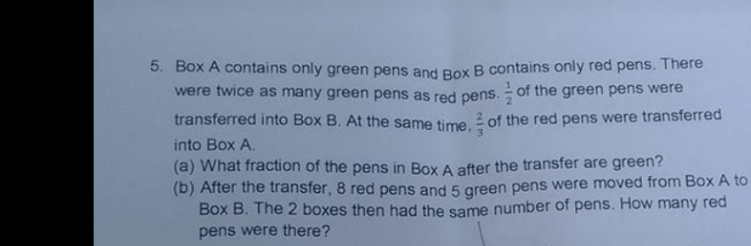# Question(a)
Before —
Box A —
green : 6 units

Box B —
red : 3 units

After —
Box A —
green : 6 units – 3 units = 3 units
red : 2 units

Box B —
red : 3 units – 2 units = 1 unit
green : 3 units

3/(3 + 2) = 3/5 of the pens in Box A after the transfer were green

(b)
(3 units – 5) + (2 units – 8) = 5 units – 13 = (1 unit + 8) + (3 units + 5) = 4 units + 13
1 unit = (13 + 13)/(5 – 4) = 26
3 units = 3 x 26 = 78

Ans : (a) 3/5 of the pens in Box A after the transfer were green; (b) 78 red pens.

0 Replies 0 Likes